Register for Maths, Science, English, Reasoning Olympiad Exams Register here | Book Free Demo for Live Olympiad Classes here | Check Olympiad Exam Dates here | Buy Practice Papers for IMO, IOM, HEO, IOEL etc here | Login here to participate in all India free mock test on every Saturday

# Numerals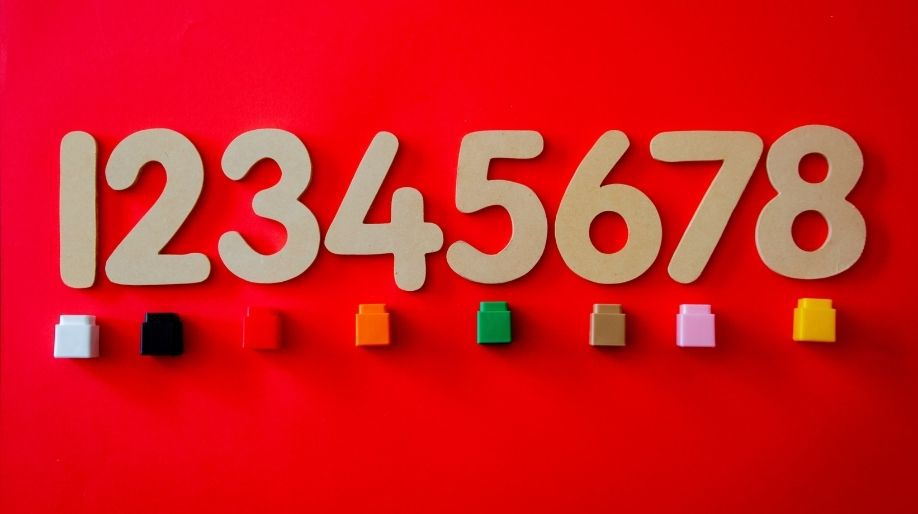What are numerals? We all come across various kinds of values which include numbers, characters, alphabets, symbols etc. But here we will specially learn about those magical figures called numbers which is the base of Mathematics and have made this subject so much interesting. Let’s find out.

• Any figure or symbol representing a number is a numeral.
• The numbers from 0 to 9 when combined and written in a specific order creates a numeral.
• The numeral can be single digit like 5 or 2 digits like 12 or 3 digits like 564 and 4 digits like 5854.

There are two Types of numerals which we should learn

• The western Arabic numerals

Example is 0,1,2,3,4,5,6,7,8,9

• The Roman Numerals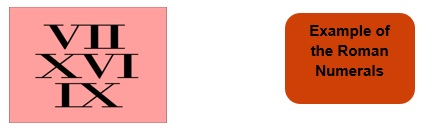Western Arabic Numerals

The numbers from 0-9 are the base of the western Arabic numerals and then their combinations which results in the formation of more numbers also come under this category.

• The numbers 0 – 9 are called as digit.
• The numbers created by their combination is numeral.

We all know two and three digit numbers and how to read and write them so here we are going to learn about the four digit numbers and how to read and write them.

Four Digit Numbers

The numeral containing four digits is called as a four digit number. For example 4586 is a four digit number.

• To identify the numbers, count the digits and hence you can predict if it is a four digit number or any other number.

Place Values

In our number system the value of a digit completely depends on its position and that position helps us to write the expanded form of that number and hence helps in identifying the number.

• Each and every number has a place value.
• We start assigning the place values from right to left.
• The place values starts from Ones then Tens, Hundreds, Thousands, Ten Thousands,

Example 1: Determine the place values of the number 24 and write its expanded form.

Solution: We will start from right most digit and then we will go to left.

Here, the right most digit is 4, hence its place value is One.

Next is 2 and hence its place value is Tens.

Therefore we can write the number as

24 = (2 x 10) + (4 x 1) = Twenty + Four = Twenty four.

Hence the expanded form is Twenty Four.

Example 2: Determine the place values of the number 789 and write its expanded form.

Solution: Here the right most digit is 9 so its place value is Ones.

Next is 8 so its place value is Tens.

Next is 7 so its place value is Hundreds.

Therefor we can write the number as

789 = (7 x 100) + (8 x 10) + (9 x 1) = 700 + 80 + 9

Which will be Seven Hundred + Eighty + Nine which makes it Seven hundred eighty nine.

Let us learn how to write these four digits numbers:

Let’ s take any 4 digit number for example we will take 1234.

Now here we know that 4 is called at ones place and 3 is at tens, 2 is at hundreds and 1 is at thousands.

So the number 1234 can be written as

1234 = (1 x 1000) + (2 x 100) + (3 x 10) + (4 x 1)

= One thousand + Two hundred + Thirty + Four

Hence the number is One thousand two hundred thirty four.

• The smallest 4 digit number is 1000 = One thousand
• The Largest 4 digit number is 9999 = Nine thousand nine hundred ninety nine.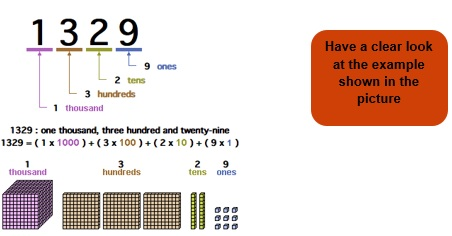Roman Numerals

The numbers from 0 – 9 and also their magical combinations can also represented using certain alphabets and this representation is called Roman Numerals.

Let us learn how to write and read roman Numerals from this table: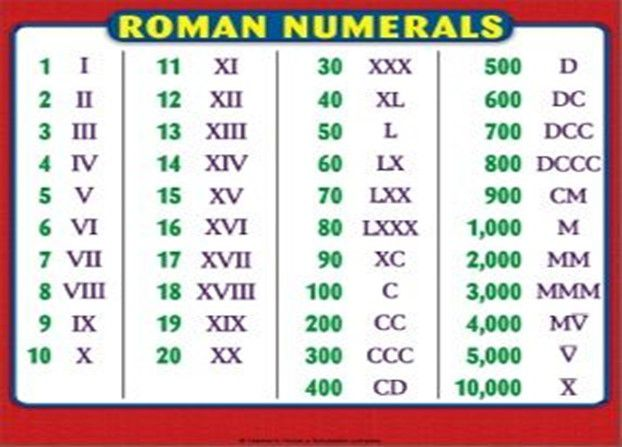Here we have to learn the representations of the numbers and then we can write the roman numerals also.

Example 3: Write 23 in Roman numeral form.

Solution: Now in 23, 3 is at ones place and 2 is at tens place.

So, 23 = (2 x 10) + (3 x 1) = 20 + 3 = XXIII

From here we learnt that we can write the roman form of any number if we know the place values and roman forms of initial numbers.

Example 4: Write the roman form of 2356.

Solution: Here 6 is at ones place, 5 is at tens, 3 is at hundreds, 2 is at thousands.

2356 = (2 x 1000) + (2 x 100) + (5 x 10) + (6 x 1)

= Two thousand + two hundred + fifty + six = MMCCLVI

Isn’t it interesting to find roman numbers and place values so let’s practice a few more questions.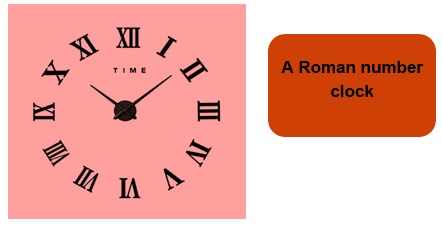Practice Questions

Q1) Count the number of 4 digit numbers among the following:

784, 45, 1235, 6547, 7895, 564, 1254, 56879, 45641, 454, 6985.

Q2) Write the expanded form of following numbers:

1. 124
2. 1654
3. 7100
4. 1100
5. 78

Q3) Find the place values of the following numbers:

1. 456
2. 523
3. 1569
4. 2307

Q4) Write the roman representation of the following numbers:

1. 145
2. 325
3. 894
4. 1006
5. 4589

Q5) State True or False:

1. Every digit has a place value.
2. We start from left most digit while assigning the place values
3. Hundreds then tens then ones.
4. 10000 is a 4 digit number
5. Largest 3 digit number is 999.

Recap

• Any figure representing a number is a numeral.
• While assigning the place values we start from right to left.
• First comes ones then tens, then hundreds, then thousands.
• By counting the digits we can predict whether the number is two or three for digit number.
• In roman numerals the numbers are just represented using the alphabets.
• The numbers from 0 to 9 are called western Arabic numerals.

## Quiz for Numerals

 Q.1 Fill in the blank. 445, 452, 459, ______, 473. a) 445 b) 480 c) 460 d) 466
 Q.2 In 7,86,452 which number is present in hundreds place? a) 4 b) 5 c) 6 d) 7
 Q.3 In 45,782 which number is present in thousands place? a) 4 b) 5 c) 7 d) 8
 Q.4 Which number has 8 in its hundreds place? a) 6,008 b) 46,782 c) 3,65,888 d) 8,88, 567
 Q.5 Find the greatest number we can make using digits 3, 2, 6 and 7. a) 7263 b) 6723 c) 7362 d) 7632
 Q.6 Find the lowest number we can make using the digits 4, 5, 2 and 8. a) 2548 b) 2458 c) 2854 d) 2845
 Q.7 Find the greatest number we can make using digits 9 and 7. (Each number is allowed to be repeated maximum twice) a) 797 b) 977 c) 997 d) 979
 Q.8 Find the lowest number we can make using digits 4 and 5. (Each number is allowed to be repeated maximum twice) a) 545 b) 544 c) 454 d) 445
 Q.9 In morning a man noticed he has 789 messages in his phone. At the end of the day he noticed he has 1008 messages. How many new messages he has received in that day? a) 199 b) 219 c) 329 d) 209
 Q.10 In a farm there are 7000 hens, the owner brings 2567 new hens. Total how many hens are there now? a) 9567 b) 7567 c) 10567 d) 2567